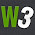## Jun 16, 2014

### C Programming #13: Operators - Increment Decrement

Even though increment and decrement operator is type of Arithmetic operator. But i think it needs special attention hence this exclusive article covering only increment and decrement operator.

## Increment (++)

'++' is the increment operator in C. As name suggests ++ increments the value by 1. It can apply only to variables, it cannot be applied to constants. There are two types of increment operator pre-increment and post-increment.

Both pre-increment and post-increment increases the value of variable by 1. Difference comes when it is used in expression. Following program will tell the difference and similarities between them. Variable 'a' will be applied with post-increment and variable 'b' will applied with pre-increment.

#include <stdio.h>
int main()
{
int a = 10;
int b = 10;

int c;
int d;

a++;
++b;

printf("Value of a and b is %d and %d\n" , a, b);

c = a++;
d = ++b;

printf("Value of a,b,c and d is %d,%d,%d and %d\n" , a, b, c, d);

return 0;
}


Output of the above program is

Value of a and b is 11 and 11
Value of a,b,c and d is 12,12,11 and 12


In first usage of a++ and ++b there is no difference between pre and post both of increment the variable by 1.

In second usage c = a++; a++ return the value of a first and later increments hence the name post.
d = ++b; here b is incremented first then returned the value hence the name pre.

## Decrement (--)

-- is the decrement operator in C. As name suggests -- decrements the value by 1. Decrement also has pre and post decrement just like increment. Hence repeating would not add any more information.

1.